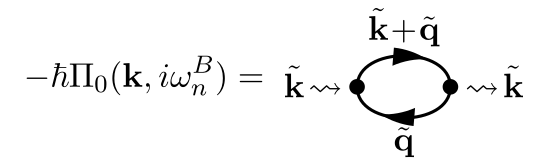Categories: Physics, Quantum mechanics.

Random phase approximation

Recall that the self-energy $$\Sigma$$ is defined as a sum of Feynman diagrams, which each have an order $$n$$ equal to the number of interaction lines. We consider the self-energy in the context of jellium, so the interaction lines $$W$$ represent Coulomb repulsion, and we use imaginary time.

Let us non-dimensionalize the Feynman diagrams in the self-energy, by measuring momenta in units of $$\hbar k_F$$, and energies in $$\epsilon_F = \hbar^2 k_F^2 / (2 m)$$. Each internal variable then gives a factor $$k_F^5$$, where $$k_F^3$$ comes from the 3D momentum integral, and $$k_F^2$$ from the energy $$1 / \beta$$:

\begin{aligned} \frac{1}{(2 \pi)^3} \int_{-\infty}^\infty \frac{1}{\hbar \beta} \sum_{n = -\infty}^\infty \cdots \:\dd{\vb{k}} \:\:\sim\:\: k_F^5 \end{aligned}

Meanwhile, every line gives a factor $$1 / k_F^2$$. The Matsubara Green’s function $$G^0$$ for a system with continuous translational symmetry is found from equation-of-motion theory:

\begin{aligned} W(\vb{k}) = \frac{e^2}{\varepsilon_0 |\vb{k}|^2} \:\:\sim\:\: \frac{1}{k_F^2} \qquad \qquad G_s^0(\vb{k}, i \omega_n^F) = \frac{1}{i \hbar \omega_n^F - \varepsilon_\vb{k}} \:\:\sim\:\: \frac{1}{k_F^2} \end{aligned}

An $$n$$th-order diagram in $$\Sigma$$ contains $$n$$ interaction lines, $$2n\!-\!1$$ fermion lines, and $$n$$ integrals, so in total it evolves as $$1 / k_F^{n-2}$$. In jellium, we know that the electron density is proportional to $$k_F^3$$, so for high densities we can rest assured that higher-order terms in $$\Sigma$$ converge to zero faster than lower-order terms.

However, at a given order $$n$$, not all diagrams are equally important. In a given diagram, due to momentum conservation, some interaction lines carry the same momentum variable. Because $$W(\vb{k}) \propto 1 / |\vb{k}|^2$$, small $$\vb{k}$$ make a large contribution, and the more interaction lines depend on the same $$\vb{k}$$, the larger the contribution becomes.

In other words, each diagram is dominated by contributions from the momentum carried by the largest number of interactions. At order $$n$$, there is one diagram where all $$n$$ interactions carry the same momentum, and this one dominates all others at this order.

The random phase approximation consists of removing most diagrams from the defintion of the full self-energy $$\Sigma$$, leaving only the single most divergent one at each order $$n$$, i.e. the ones where all $$n$$ interaction lines carry the same momentum and energy:Where we have defined the screened interaction $$W^\mathrm{RPA}$$, denoted by a double wavy line:Rearranging the above sequence of diagrams quickly leads to the following Dyson equation:In Fourier space, this equation’s linear shape means it is algebraic, so we can write it out:

\begin{aligned} \boxed{ W^\mathrm{RPA} = W + W \Pi_0 W^\mathrm{RPA} } \end{aligned}

Where we have defined the pair-bubble $$\Pi_0$$ as follows, with an internal wavevector $$\vb{q}$$, fermionic frequency $$i \omega_m^F$$, and spin $$s$$. Abbreviating $$\tilde{\vb{k}} \equiv (\vb{k}, i \omega_n^B)$$ and $$\tilde{\vb{q}} \equiv (\vb{q}, i \omega_n^F)$$:We isolate the Dyson equation for $$W^\mathrm{RPA}$$, which reveals its physical interpretation as a screened interaction: the “raw” interaction $$W \!=\! e^2 / (\varepsilon_0 |\vb{k}|^2)$$ is weakened by a term containing $$\Pi_0$$:

\begin{aligned} W^\mathrm{RPA}(\vb{k}, i \omega_n^B) = \frac{W(\vb{k})}{1 - W(\vb{k}) \: \Pi_0(\vb{k}, i \omega_n^B)} = \frac{e^2}{\varepsilon_0 |\vb{k}|^2 - e^2 \Pi_0(\vb{k}, i \omega_n^B)} \end{aligned}

Let us evaluate the pair-bubble $$\Pi_0$$ more concretely. The Feynman diagram translates to:

\begin{aligned} -\hbar \Pi_0(\vb{k}, i \omega_n^B) &= - \sum_{s} \frac{1}{(2 \pi)^3} \int \frac{1}{\hbar \beta} \sum_{m = -\infty}^\infty \hbar G_s(\vb{k} \!+\! \vb{q}, i \omega_n^B \!+\! i \omega_m^F) \: \hbar G_s(\vb{q}, i \omega_m^F) \dd{\vb{q}} \\ &= - \frac{2 \hbar}{(2 \pi)^3} \int \frac{1}{\beta} \sum_{m = -\infty}^\infty \frac{1}{i \hbar \omega_n^B + i \hbar \omega_m^F - \varepsilon_{\vb{k}+\vb{q}}} \: \frac{1}{i \hbar \omega_m^F - \varepsilon_{\vb{q}}} \dd{\vb{q}} \end{aligned}

Here we recognize a Matsubara sum, and rewrite accordingly. Note that the residues of $$n_F$$ are $$1 / (\hbar \beta)$$ when it is a function of frequency, and $$1 / \beta$$ when it is a function of energy, so:

\begin{aligned} \Pi_0(\vb{k}, i \omega_n^B) &= \frac{2}{(2 \pi)^3} \int \frac{n_F(\varepsilon_{\vb{k}+\vb{q}} - i \hbar \omega_n^B)}{(\varepsilon_{\vb{k}+\vb{q}} - i \hbar \omega_n^B) - \varepsilon_{\vb{q}}} + \frac{n_F(\varepsilon_{\vb{q}})}{i \hbar \omega_n^B + (\varepsilon_{\vb{q}}) - \varepsilon_{\vb{k}+\vb{q}}} \dd{\vb{q}} \\ &= \frac{2}{(2 \pi)^3} \int \frac{n_F(\varepsilon_{\vb{q}}) - n_F(\varepsilon_{\vb{k}+\vb{q}})} {i \hbar \omega_n^B + \varepsilon_{\vb{q}} - \varepsilon_{\vb{k}+\vb{q}}} \dd{\vb{q}} \end{aligned}

Where we have used that $$n_F(\varepsilon \!+\! i \hbar \omega_n^B) = n_F(\varepsilon)$$. Analogously to extracting the retarded Green’s function $$G^R(\omega)$$ from the Matsubara Green’s function $$G^0(i \omega_n^F)$$, we replace $$i \omega_n^F \to \omega \!+\! i \eta$$, where $$\eta \to 0^+$$ is a positive infinitesimal, yielding the retarded pair-bubble $$\Pi_0^R$$:

\begin{aligned} \boxed{ \Pi_0^R(\vb{k}, \omega) = \frac{2}{(2 \pi)^3} \int \frac{n_F(\varepsilon_{\vb{q}}) - n_F(\varepsilon_{\vb{k}+\vb{q}})} {\hbar (\omega + i \eta) + \varepsilon_{\vb{q}} - \varepsilon_{\vb{k}+\vb{q}}} \dd{\vb{q}} } \end{aligned}

This is as far as we can go before making simplifying assumptions. Therefore, we leave it at:

\begin{aligned} \boxed{ W^\mathrm{RPA}(\vb{k}, \omega) = \frac{e^2}{\varepsilon_0 |\vb{k}|^2 - e^2 \Pi_0(\vb{k}, \omega)} } \end{aligned}

1. H. Bruus, K. Flensberg, Many-body quantum theory in condensed matter physics, 2016, Oxford.

© Marcus R.A. Newman, a.k.a. "Prefetch". Available under CC BY-SA 4.0.